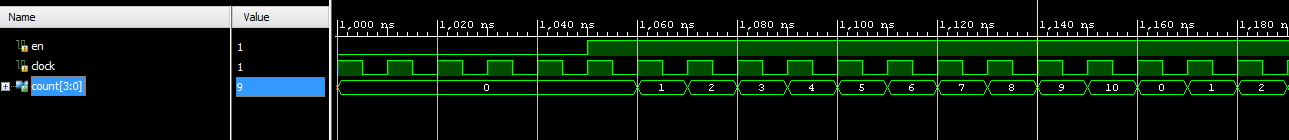# Verilog Code Decade Counter | How to Design Decade Counter in Verilog

#### Verilog Implementation of Decade Counter

In this post we are going to share the verilog code of decade counter. As we you know, decade counter is a counter that counts from 0 to 9.  Here we are implementing it in HDL such as verilog. The verilog implementation of Decade Counter is given below.

```module decade_counter(en, clock, count);
input en, clock;
output reg [3:0] count;

always @( posedge clock)
begin
if(en)
begin

if ( count>=4'd0 && count<4'd10)
count<=count+4'd1;

else
count<=4'd0;

end

else
count<=4'd0;
end
endmodule```

##### The Testbench is given below.
````timescale 1ns/1ps
wire [3:0] count;
reg en,clock;

initial begin
\$display(\$time, " << Starting the Simulation >>");
en=0;
clock=0;
#20 en=1'd1;
end

always
#5 clock=~clock;

initial
\$monitor ( \$time , "clock= %b, count= %d, en= %b",    clock,count, en);

endmodule```

### The simulation result of decade counter verilog code is given below.```module decade_counter ( output reg [3:0] q,
input clk );
always @(posedge clk)
q <= q = = 9 ? 0 : q + 1;
endmodule```